# Initialize Classification Model (SVM) VI

LabVIEW 2018 Analytics and Machine Learning Toolkit Help

Edition Date: July 2018

Part Number: 377059B-01

»View Product InfoDownload Help (Windows Only)

Owning Palette: Classification VIs

Requires: Analytics and Machine Learning Toolkit

Initializes the hyperparameters of the support vector machine (SVM) algorithm. You can either directly set the hyperparameters or specify multiple values for each hyperparameter. If you specify multiple values for each hyperparameter, the Train Classification Model VI uses grid search to find the optimal set of hyperparameters.

Examples

Use the pull-down menu to select an instance of this VI.

 Select an instance Set ParametersSearch Parameters

## Set Parameters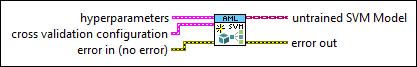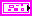hyperparameters specifies the hyperparameters of the SVM model.SVM type specifies the type of the SVM model.

 1 C_SVC (default)—Specifies the C-SVM model. 2 NU_SVC—Specifies the nu-SVM model.c specifies the c parameter for the C-SVM model. The default is 1. The lower the value of c, the more wrongly classified training samples the SVM model allows for. The c parameter must be greater than 0. This input is valid only if SVM type is C_SVC.nu specifies the nu parameter for the nu-SVM model. The nu parameter is both a lower bound for the number of samples that are support vectors and an upper bound for the number of samples that are on the wrong side of the hyperplane. The default is 0.5. The nu parameter must be in the range [0,1]. This input is valid only if SVM type is NU_SVC.

For example, if nu is 0.05, the training samples that are wrongly classified are not allowed to take up more than 5 percent of all training samples. Also, at least 5 percent of the training samples are support vectors.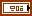kernel settings specifies settings to configure the kernel function.

This VI supports the following kernel functions:

Kernel functionDefinitionDescription
Linear function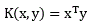x and y are the input sample vectors.
Polynomial function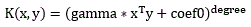x and y are the input sample vectors; gamma, coef0, and degree are the algorithm coefficients.
Radial basis function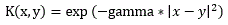x and y are the input sample vectors.
Sigmoid functionx and y are the input sample vectors; gamma and coef0 are the algorithm coefficients.type specifies the type of the kernel function.

 0 Linear (default)—Linear function 1 Polynomial—Polynomial function 2 RBF—Radial basis function 3 Sigmoid—Sigmoid function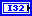degree specifies the degree coefficient of the kernel function. The default is 3.gamma specifies the gamma coefficient of the kernel function. The default is 1.coef0 specifies the coef0 coefficient of the kernel function. The default is 0.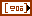weighted c specifies different weights of parameter c for different classes in the training data. Use this input when the training data is unbalanced. In unbalanced data, the number of samples for some classes is much greater than that in other classes. If you specify this input, the Deploy Classification Model VI cannot return the predicted probabilities.label specifies the label of the data to assign weight.weight specifies the weight to assign to the label.tolerance specifies the tolerance for the stopping criteria. The default is 0.001.max iteration specifies the maximum number of optimization iterations for the stopping criteria. The default is 100000. The model fitting stops if the number of optimization iterations reaches max iteration.cross validation configuration specifies settings for cross validation.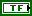enable? specifies whether this VI enables cross validation in model training. The default is FALSE, which specifies that this VI disables cross validation in model training.

If enable? is TRUE, the Train Classification Model VI can return confusion matrix and metrics.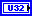number of folds specifies the number of sections that this VI divides the training data into. The default is 3. One section is test data, and the remaining sections are training data. The Evaluate Classification Model VI repeats the cross-validation process for n times, where n equals number of folds.metric configuration specifies the configuration for the evaluation metric in cross validation.average method specifies the averaging method for this VI to calculate metric values for multiclass classification.

 0 Micro (default)—Calculates metric values for each sample and returns the mean of the metric values for all samples. 1 Macro—Calculates the metric values for each label and returns the mean of the metric values for all labels. 2 Weighted—Calculates the metric values for each label and returns the mean of weighted metric values for all labels. The number of true cases in a label determines the weight of the metric value of the label. 3 Binary—Calculates the metric values for the class that positive label specifies.positive label specifies the label of the class to calculate metric values. The default is 0. This input is valid only if average method is Binary.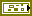error in describes error conditions that occur before this node runs. This input provides standard error in functionality.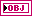untrained SVM model returns the initialized SVM model for training.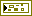error out contains error information. This output provides standard error out functionality.

## Search Parameters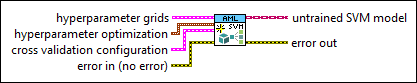hyperparameter grids specifies multiple values for each hyperparameter of the SVM model.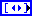SVM type specifies the type of the SVM model.

 1 C_SVC (default)—Specifies the C-SVM model. 2 NU_SVC—Specifies the nu-SVM model.c specifies the c parameter for the C-SVM model. The lower the value of c, the more wrongly classified training samples the SVM model allows for. The c parameter must be greater than 0. This input is valid only if SVM type is C_SVC.nu specifies the nu parameter for the nu-SVM model. The nu parameter is both a lower bound for the number of samples that are support vectors and an upper bound for the number of samples that are on the wrong side of the hyperplane. The nu parameter must be in the range [0,1]. This input is valid only if SVM type is NU_SVC.

For example, if nu is 0.05, the training samples that are wrongly classified are not allowed to take up more than 5 percent of all training samples. Also, at least 5 percent of the training samples are support vectors.kernel settings specifies settings to configure the kernel function.

This VI supports the following kernel functions:

Kernel functionDefinitionDescription
Linear functionx and y are the input sample vectors.
Polynomial functionx and y are the input sample vectors; gamma, coef0, and degree are the algorithm coefficients.
Radial basis functionx and y are the input sample vectors.
Sigmoid functionx and y are the input sample vectors; gamma and coef0 are the algorithm coefficients.type specifies the type of the kernel function.

 0 Linear—Linear function 1 Polynomial—Polynomial function 2 RBF—Radial basis function 3 Sigmoid—Sigmoid function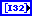degree specifies the degree coefficient of the kernel function.gamma specifies the gamma coefficient of the kernel function.coef0 specifies the coef0 coefficient of the kernel function.weighted c specifies different weights of parameter c for different classes in the training data. Use this input when the training data is unbalanced. In unbalanced data, the number of samples for some classes is much greater than that in other classes. If you specify this input, the Deploy Classification Model VI cannot return the predicted probabilities.label specifies the label of the data to assign weight.weight specifies the weight to assign to the label.tolerance specifies the tolerance for the stopping criteria. The default is 0.001.max iteration specifies the maximum number of optimization iterations for the stopping criteria. The default is 100000. The model fitting stops if the number of optimization iterations reaches max iteration.hyperparameter optimization specifies the method of optimization to determine the optimal hyperparameter settings.hyperparameter search method specifies the method to search for the optimal set of hyperparameters.

 0 Exhaustive search (default)—Tests all possible hyperparameter combinations in the training process. This method is reliable but time-consuming. 1 Random search—Tests a random subset of the hyperparameter combinations in the training process. This method is faster.number of searchings specifies the number of hyperparameter combinations that this VI selects to train the model. The default is 1. This input is valid only if hyperparameter search method is Random search.evaluation metric specifies the metric to evaluate the trained model with different hyperparameter combinations. The Train Classification Model VI finds the optimal hyperparameter set based on evaluation metric.

 0 Accuracy 1 Precision 2 Recall 3 F1 scorecross validation configuration specifies settings for cross validation.number of folds specifies the number of sections that this VI divides the training data into. The default is 3. One section is test data, and the remaining sections are training data. The Evaluate Classification Model VI repeats the cross-validation process for n times, where n equals number of folds.metric configuration specifies the configuration for the evaluation metric in cross validation.average method specifies the averaging method for this VI to calculate metric values for multiclass classification.

 0 Micro (default)—Calculates metric values for each sample and returns the mean of the metric values for all samples. 1 Macro—Calculates the metric values for each label and returns the mean of the metric values for all labels. 2 Weighted—Calculates the metric values for each label and returns the mean of weighted metric values for all labels. The number of true cases in a label determines the weight of the metric value of the label. 3 Binary—Calculates the metric values for the class that positive label specifies.positive label specifies the label of the class to calculate metric values. The default is 0. This input is valid only if average method is Binary.error in describes error conditions that occur before this node runs. This input provides standard error in functionality.untrained SVM model returns the initialized SVM model for training.error out contains error information. This output provides standard error out functionality.

## Examples

Refer to the following VIs for examples of using the Initialize Classification Model (SVM) VI:

• Classification (Set Parameters, Training) VI: labview\examples\AML\Classification
• Classification (Search Parameters, Training) VI: labview\examples\AML\Classification